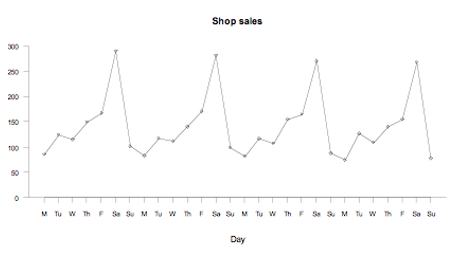Te Kete Ipurangi
Communities
Schools

# Glossary page L

A  B  C  D  E  F G  H  I J K  L  M  N  O  P  Q  R  S  T  U  V  W X Y Z

## Least-squares regression line

The most common method of choosing the line that best summarises the linear relationship (or linear trend) between the two variables in a linear regression analysis, from the bivariate data collected.

Of the many lines that could usefully summarise the linear relationship, the least-squares regression line is the one line with the smallest sum of the squares of the residuals.

Two other properties of the least-squares regression line are:

1. The sum of the residuals is zero.
2. The point with x-coordinate equal to the mean of the x-coordinates of the observations and with y-coordinate equal to the mean of the y-coordinates of the observations is always on the least-squares regression line.

### Curriculum achievement objectives reference

Statistical investigation: Level (8)

## Likelihood

The notion of an outcome being probable. Likelihood is sometimes used as a simpler alternative to probability.

In a situation involving elements of chance, equal likelihoods mean that, for any trial, each outcome has the same chance of occurring.

Similarly, different likelihoods mean that, for any trial, not all of the outcomes have the same chance of occurring.

### Curriculum achievement objectives reference

Probability: Level 2

## Line graph

A graph, often used for displaying time-series data, in which a series of points representing individual observations are connected by line segments.

Line graphs are useful for showing changes in a variable over time.

### Example

Daily sales, in thousands of dollars, for a hardware store were recorded for 28 days. These data are displayed on the line graph below.If you cannot view or read this graph, select this link to open a text version.

### Curriculum achievement objectives references

Statistical investigation: Levels (3), (4), (5), (6), (7), (8)

## Linear combination (of random variables)

A way of combining two or more random variables in which each random variable is multiplied by a number and all of these are then added.

### Example

If X and Y are random variables then T = 2X + 3Y is a linear combination of X and Y.

### Curriculum achievement objectives reference

Probability: Level 8

## Linear regression

A form of statistical analysis that uses bivariate data (where both are numerical variables) to examine how knowledge of one of the variables (the explanatory variable) provides information about the values of the other variable (the response variable). The roles of the explanatory and response variables are therefore different.

When the bivariate numerical data are displayed on a scatter plot, the relationship between the two variables becomes visible. Linear regression fits a straight line to the data that is added to the scatter plot. The fitted line helps to show whether a linear regression model is a good fit to the data.

If a linear regression model is appropriate, then the fitted line (regression line) is used to predict a value of the response variable for a given value of the explanatory variable and to describe how the values of the response variable change, on average, as the values of the explanatory variable change.

An appropriately fitted linear regression model estimates the true, but unknown, linear relationship between the two variables and the underlying system the data was taken from is regarded as having two components: trend (the general linear tendency) and scatter (variation from the trend).

Note: Linear regression can be used when there is more than one explanatory variable, but at level 8 only one explanatory variable is used. When there is one explanatory variable, the method is called simple linear regression.

### Curriculum achievement objectives reference

Statistical investigation: Level 8

## Linear transformation (of a random variable)

A change to a random variable made by one or more of the following operations:

• adding or subtracting a number
• multiplying or dividing by a number.

### Example

If X is a random variable then T = 2X + 3 is a linear transformation of X.

### Curriculum achievement objectives reference

Probability: Level 8

## Lower quartile

See: quartiles

### Curriculum achievement objectives references

Statistical investigation: Levels (5), (6), (7), (8)

Last updated October 16, 2013